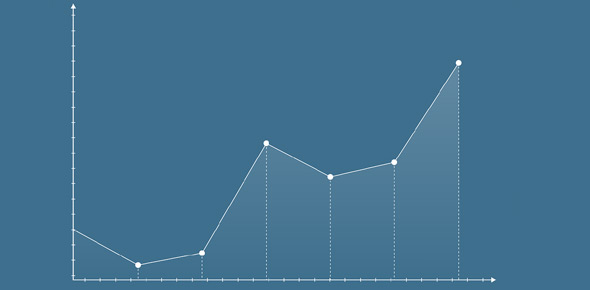2620 Scatter Plots Transformation Circle In A Minute

8 Questions | Total Attempts: 64SettingsSee how quickly you can complete these questions about the circle.

• 1.
Which transformations apply in Quadrant 1
• A.

X squared

• B.

Y squared

• C.

Reciprocal x

• D.

Reciprocal y

• E.

Log x

• F.

Log y

• 2.
Which transformations apply in Quadrant 2
• A.

X squared

• B.

Y squared

• C.

Reciprocal x

• D.

Reciprocal y

• E.

Log x

• F.

Log y

• 3.
Which transformations apply in Quadrant 3
• A.

X squared

• B.

Y squared

• C.

Reciprocal x

• D.

Reciprocal y

• E.

Log x

• F.

Log y

• 4.
Which transformations apply in Quadrant 4
• A.

X squared

• B.

Y squared

• C.

Reciprocal x

• D.

Reciprocal y

• E.

Log x

• F.

Log y

• 5.
What is the function of the squared transformations?
• A.

To stretch values

• B.

To compress values

• C.

To compress very large values

• D.

To take over the universe

• 6.
What is the function of the log transformations?
• A.

To stretch values

• B.

To compress values

• C.

To compress very large values

• D.

To compress the Decepticons

• 7.
What is the function of the reciprocal transformations?
• A.

To stretch values

• B.

To compress values

• C.

To compress very large values

• D.

To compress the Decepticons

• 8.
What is the function of the transformer Bumblebee?
• A.

To stretch values

• B.

To compress values

• C.

To compress very large values

• D.

To fight the Decepticons

Related TopicsBack to top